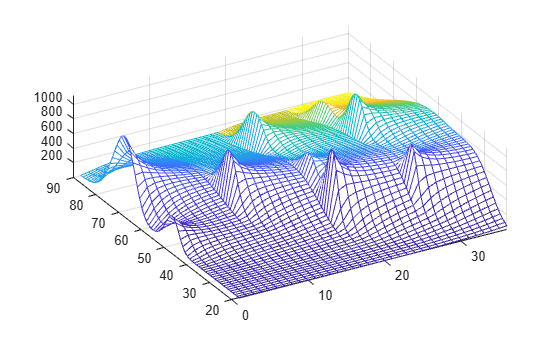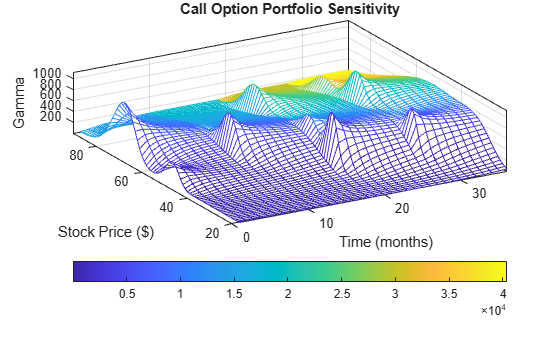# Plotting Sensitivities of a Portfolio of Options

This example plots gamma as a function of price and time for a portfolio of ten Black-Scholes options.

The plot in this example shows a three-dimensional surface. For each point on the surface, the height (z-value) represents the sum of the gammas for each option in the portfolio weighted by the amount of each option. The x-axis represents changing price, and the y-axis represents time. The plot adds a fourth dimension by showing delta as surface color. This example has applications in hedging. First set up the portfolio with arbitrary data. Current prices range from \$20 to \$90 for each option. Then, set the corresponding exercise prices for each option.

```Range = 20:90; PLen = length(Range); ExPrice = [75 70 50 55 75 50 40 75 60 35];```

Set all risk-free interest rates to 10%, and set times to maturity in days. Set all volatilities to 0.35. Set the number of options of each instrument, and allocate space for matrices.

```Rate = 0.1*ones(10,1); Time = [36 36 36 27 18 18 18 9 9 9]; Sigma = 0.35*ones(10,1); NumOpt = 1000*[4 8 3 5 5.5 2 4.8 3 4.8 2.5]; ZVal = zeros(36, PLen); Color = zeros(36, PLen);```

For each instrument, create a matrix (of size `Time` by `PLen`) of prices for each period.

```for i = 1:10 Pad = ones(Time(i),PLen); NewR = Range(ones(Time(i),1),:);```

Create a vector of time periods `1` to `Time` and a matrix of times, one column for each price.

``` T = (1:Time(i))'; NewT = T(:,ones(PLen,1));```

Use the Black-Scholes gamma and delta sensitivity functions `blsgamma` and `blsdelta` to compute gamma and delta.

``` ZVal(36-Time(i)+1:36,:) = ZVal(36-Time(i)+1:36,:) ... + NumOpt(i) * blsgamma(NewR, ExPrice(i)*Pad, ... Rate(i)*Pad, NewT/36, Sigma(i)*Pad); Color(36-Time(i)+1:36,:) = Color(36-Time(i)+1:36,:) ... + NumOpt(i) * blsdelta(NewR, ExPrice(i)*Pad, ... Rate(i)*Pad, NewT/36, Sigma(i)*Pad); end```

Draw the surface as a mesh, set the viewpoint, and reverse the x-axis because of the viewpoint. The axes range from `20` to `90`, `0` to `36`, and -∞ to ∞.

```mesh(Range, 1:36, ZVal, Color); view(60,60); set(gca, 'xdir','reverse', 'tag', 'mesh_axes_3'); axis([20 90 0 36 -inf inf]);```Add a title and axis labels and draw a box around the plot. Annotate the colors with a bar and label the color bar.

```title('Call Option Portfolio Sensitivity'); xlabel('Stock Price (\$)'); ylabel('Time (months)'); zlabel('Gamma'); set(gca, 'box', 'on'); colorbar('horiz');```